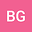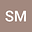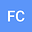Impact of changing concavity indices on channel steepness and divide migration metrics
•••• +2## Abstract

The concavity index, $\theta$, describes how quickly river channel gradient declines downstream. It is used in calculations of normalized channel steepness index, $k_{sn}$, a metric for comparing the relative steepness of channels with different drainage area. It is also used in calculating a transformed longitudinal coordinate, $\chi$, which has been employed to search for migrating drainage divides. Here we quantify the variability in $\theta$ across multiple landscapes distributed across the globe. We describe the degree to which both the spatial distribution and magnitude of $k_{sn}$ and $\chi$ can be distorted if $\theta$ is assumed, not constrained. Differences between constrained and assumed $\theta$ of 0.1 or less are unlikely to affect the spatial distribution and relative magnitude of $k_{sn}$ values, but larger differences can change the spatial distribution of $k_{sn}$ and in extreme cases invert differences in relative steepness: relatively steep areas can appear relatively gentle areas as quantified by $k_{sn}$. These inversions are function of the range of drainage area in the considered watersheds. We also demonstrate that the $\chi$ coordinate, and therefore the detection of migrating drainage divides, is sensitive to varying values of $\theta$. The median of most likely $\theta$ across a wide range of mountainous and upland environments is 0.425, with first and third quartile values of 0.225 and 0.575. This wide range of variability suggests workers should not assume any value for $\theta$, but should instead calculate a representative $\theta$ for the landscape of interest, and exclude basins for which this value is a poor fit.
Oct 2021Published in Journal of Geophysical Research: Earth Surface volume 126 issue 10. 10.1029/2020JF006060